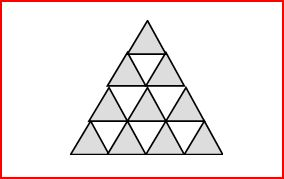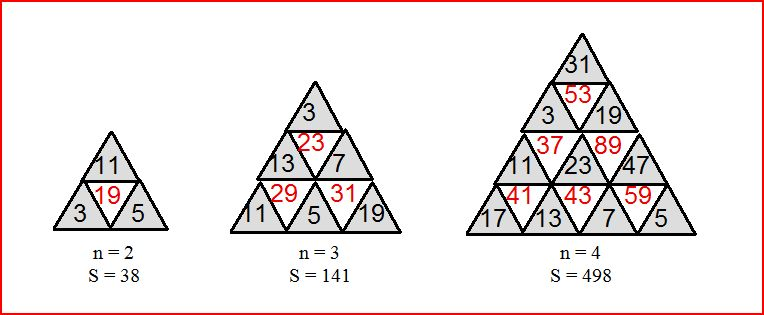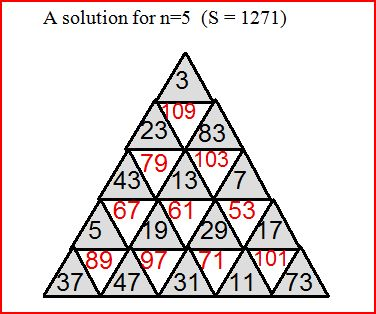Problems & Puzzles: Puzzles

Puzzle 579.- A triangular array with primes

Jean Brette sent the following nice puzzle:A triangle is divided in n^2 small triangles, and colored like a chessboard. (here n = 4)

Fill it with distinct primes with the following rule : A prime in a white triangle is equal to the sum of his three neighbors, in the grey triangles. Examples  (S is the sum of the primes used)Q1. Could you find similar triangles for   n > 5 ?  (with the smallest S, if possible)
Q2. Does it exist a solution for each n ?

Jean Brette himself found one solution using consecutive primes! here is it:

n=3, S=127, Consecutive primes

17
7 29 5
13 23 3 19 11

Q3. Does it exist solutions with consecutive primes  for n>3?

Q4. Does it exist solutions using consecutive integers?

Contributions came from Jean Brette (BTW, all of his solutions came without using a PC), Hakan Summakoğlu & Robert D. Mohr.

***

Jean Brette wrote:

Q3. n=4, S= 438, Consecutive primes!!!

5

13 41 23
7
37 17 43  3
29
47 11 59 31 53 19

Q4.

There is no solution with consecutive and positive integers starting with  1- You find the proof attached.

But if you can use zero or negative numbers:

1) If we can use the  zero, here are  solutions  with  n = 2 and  n = 3

2

0   3   1
--------------------------

2

0    5    3

6    7    1    8    4

2) if we can use negative numbers, here  are solutions with  n = 2 and  n = 3

2

0   1   -1

------------------------
With  -2, -1, 0, 1, 2, 3, 4, 5, 6

3

4    5    -2

2    6    0    -1    1

With  -3, - 2, -1, 0, 1, 2, 3, 4, 5

-2

4    5    3

-3    1    0    2    -1

***

Hakan wrote:

Q1:

 n=6,S=3116 3 5 19 11 7 29 17 41 13 23 61 31 101 53 103 37 43 113 47 137 59 179 67 193 89 109 223 71 191 73 211 79 229 83 269 97
 n=7,S=6283 3 5 19 11 7 29 17 41 13 23 61 31 101 53 103 37 43 113 47 137 59 179 67 193 89 71 197 83 227 97 229 73 271 131 359 139 157 379 151 313 79 283 107 307 127 367 109 397 149

Q3:

 n=5, S=1159, Consecutive primes 3 5 61 53 29 71 37 101 11 31 83 23 73 13 67 43 41 89 17 59 19 79 47 97 7

***

Robert wrote:

For Q4, the n=3 case a solution with lowest possible S value is S=48, an "almost-consecutive":

6
1 9 2
4
8 3 10 5

***

Robert D. Mohr wrote on March 2011:

Seven solutions for n=4 using consecutive primes:

5                                                                           5
"41"                                                                       "41"
23       13                                                               7          29
"43"     "37"                                                            "31"       "43"
3         17         7                                                    13       11          3
"53"     "59"       "47"                                                "53"     "47"      "59"
19      31        11         29                                        23       17        19         37

11                                                                         13
"59"                                                                       "47"
17       31                                                                3        31
"29"    "41"                                                            "29"      "43"
5      7        3                                                       19       7          5
"47"   "43"    "53"                                                   "59"    "41"     "53"
19     23     13      37                                              17      23     11        37

11                                                                         11
"41"                                                                      "47"
17          13                                                             5          31
"59"       "43"                                                         "29"       "41"
19         23          7                                                 17        7            3
"53'       "31"      "47"                                              "59"     "43"       "53"
29        5            3           37                                    19       23         13         37

23
"41"
11             7
"47"         "29"
17            19            3
"53"          "37"         "59"
31            5              13             43

***

Jean Brette added on March 11:

A) Congratulations to Hakan and his solution  for  Q3  and  n = 5  !!!

If he has used a computer, it will be interesting to use it again to find   ALL   the solutions for Q3 with  n= 4
My feeling tells me that they are  many.

B) Q1 ;  n = 6

My best solution is :

23

127    229    79

3    173     43    139    17

71  103     29   109      37     61      7

13    89      5    101     67    151    47    107    53

41  113   59    83      19     97      11    131   73    157     31

( among the 36 first odd primes, 149 and  137 was not used, and was replaced by  229 and 173)

So
S = 2582 + (229 + 173) – (149+137)= Sp + 116 = 2698                                where  Sp = 2682 is the sum of the 36 first odd primes.

C)   Q4 ; n = 3.

a) The minimal solution given by Robert Mohr is quite surprising, since it uses “almost consecitive and positive numbers”
So we have two more questions :

“Question 5” : What are the minimal solutions for n>3 ? (with positive integers)
“Question 6” : The numbers in these solutions are they “almost consecutive” ?

b) An answer to  Q4 and Q6; n =3

There are two , and only two, distinct solutions for n=3  (proof attached   Pz 579  Q6.doc )

5

1     8     2

6    10    3     9     4             and the  Robert’s solution.

c) Question 5, n = 4

I’ve found  three solutions with sum  S= 142.   The two first use the integers (1,2,.....13, 14,  17, 20)

The third is very nice, with only one gap (14, 15) and  (17, 18) added. So the integers are : ( 1, 2, .....12, 13,   16, 17, 18)
The ten first are grey.

8

7     18      3

2     13      4      12      5

9     17      6      11      1     16     10

Since they are “hand made”,  I don’t know if they are minimal.      :o(

D) Filling the area with positive odd numbers.  (n = 3)

With the same questions (Q5 and Q6)

Curiosly, this time, there are solutions for n = 3 with consecutive odd numbers.

7                                                                     11

5     13    1                                                      5     17     1

9     17      3     15     11            and                 7      15     3     13     9

***

OK this took about 36 hours to run. I'll have to improve the elegance of my code for n=6 and higher or it will take quite a bit longer.

Anyway, believe it or not there are 2949 solutions using consecutive primes for n=5 with S=1159.

I have enclosed a notepad document with all solutions. Note that each solution is given as a triangle with the 15 gray squares first with a second triangle showing the 10 white squares immediately below.

***Records   |  Conjectures  |  Problems  |  PuzzlesHome | Melancholia | Problems & Puzzles | References | News | Personal Page | Puzzlers | Search | Bulletin Board | Chat | Random Link Copyright © 1999-2012 primepuzzles.net. All rights reserved.